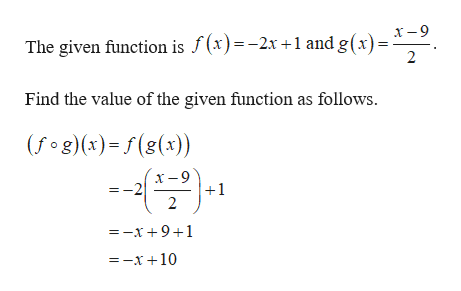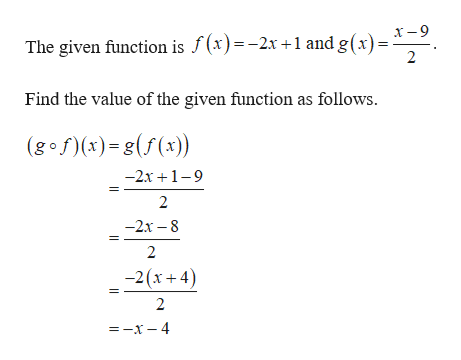# f(x) = -2x+1 and g (x)=x-9/2(a) ( f o g )(x) =(b)( g o f )(x)=(c) thus g(x) =       an inverse function of f(x)n

Question
6 views

f(x) = -2x+1 and g (x)=x-9/2

(a) ( f o g )(x) =

(b)( g o f )(x)=

(c) thus g(x) =       an inverse function of f(x)n

check_circle

Step 1

(a)

The given function:help_outlineImage TranscriptioncloseThe given function is f(x)=-2x +1 and g (x) = X-9. 2 Find the value of the given function as follows (f g (x)=f(g(x)) х -9 -2 1 2 =-x+ 9+1 =-x+10 fullscreen
Step 2

(b)

The given fun...help_outlineImage Transcriptioncloseх -9 The given function is f(x)=-2x +1 and g(x) = 2 Find the value of the given function as follows (gof(x)-g(f(x)) -2х +1-9 2 —2х — 8 2 -2(x+ 4) 2 —-х—4 fullscreen

### Want to see the full answer?

See Solution

#### Want to see this answer and more?

Solutions are written by subject experts who are available 24/7. Questions are typically answered within 1 hour.*

See Solution
*Response times may vary by subject and question.
Tagged in

### Other